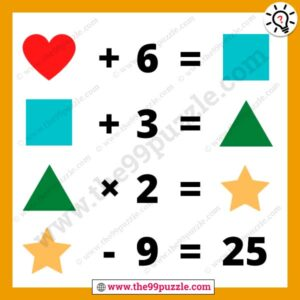# Find out each shape value with answer – Puzz249

In this logical shape picture, math reasoning can twist your brain. Can you find out each shape value brain teaser picture puzzle? In this puzzle picture, you see the four different shapes which are in mathematical logic. Online puzzles help to improve your logical mind. Most puzzlers fail to solve this brain teaser puzzle. This math picture is more tricky than other picture puzzles. If you practice more math equation puzzles, find this website and try to solve all these puzzles. To learn and solve quickly math tricks by the practice of these puzzles math. If you are smart in puzzles you can solve this answer.###### Explanation:

Love+6 = Square

⇒ 8+6 = 14

Square+3 = Triangle

⇒ 14+3 = 17

Triangle×2 = Star

⇒ 17×2 = 34

Star-9 = 25

⇒ 34-9 = 25

Love = 8

Square = 14

Triangle = 17

Star = 34

Here you will find many , and many more puzzles. We are creating various fun and interesting types of puzzles for adults and kids. Find out each shape value with the answer. Always think logically to solve easily these types of puzzles. On this website, you will play very fun games & quizzes that can improve your visual and logical thinking. Regularly we are updated with new puzzles & quizzes on this site. Check out daily to get new puzzles, riddles & quizzes.